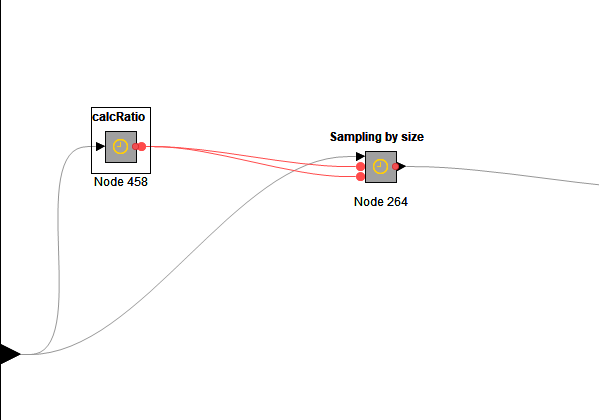# Unbalanced Data

Hi
Is there a workflow or meta-node or a node that takes data and able to sample according to a specific ratio?
For example, if the ration 1:1 then it will get the equal size if the ratio 1:2 then it will get twice examples of the minority class and so on

Malik

Hi @malik,

The Row Sampling node can do that. Use the “Stratified sampling” option and select the class column so the node will keep the (approximate) ratio.Hi @armingrudd
Thanks for your reply. I didn’t see how it will works ?
I have two-classes , neg 22 and pos 110. So how i will get neg 22 and pos 44? or neg 22 and pos 66?

Malik

It will keep the ratio between classes and sample the data based on the relative value you specify.

For example, if you have 300 data rows in total: 100 class A values and 200 class B values. Then if you take 10% of the values and use the “Stratified sampling” then you will have 30 data rows in total: 10 class A and 20 class B.

I just got what you want. I cannot remember any specific node which is able to does this task. I will build a component for this and then get back to you.

1 Like

Hi @malik

I created a workflow unbalanced.knwf (304.3 KB), that makes it possible to change the ratio between two classes. The flow can be optimized, automated but is this the direction you are looking at?

gr. Hans

2 Likes

Hey @malik,

Here is a component which takes a ratio and a class column and returns the modified ratio:

Ratio Modifier.knar (25.1 KB)

The ratio format is n:m where n must be equal or less than m (I did not test the component when n is greater than m). The smaller class would be n and the bigger class would be m. As it is obvious, this component works only on a binary class column.If you are just trying to balance your dataset then you can use the equal size sampling node.

1 Like

If this is for model building instead of discarding data I would simply use a model that allows class weights to be assigned. Admittedly this is kind of a problem in knime currently. AFAIK you can only do that with xgboost at the moment (scale_pos_weight parameter) and there is a gotcha here as well as you need to use the edit nominal domain node so that the positive class is the right one.

1 Like

I have created one using Python nodeSampleByRatio.knwf (18.6 KB)

Summarizing the answers above, the options are:

• downsampling majority classes using the aforementioned Equal Size Sampling node. But you loose information by throuwing away a part of majority-class rows
• upsampling minority class by a custom component. This will work if your data is small and simple implementations allow to handle only binary categorical features. if you have `N` classes and in extreme case the majority class is larger than alll others by factor `M`, then upsampled dataset will be `~N*M` larger, which can cause RAM problems.
• upsample minority using the SMOTE node. But this will generate artificial rows, which not always is meaningful.
• use weights in training. Indeed, this is not very wide spread in KNIME, as pointed out by @beginner, but there is the aforementioned XGBoost GBM but also H2O models allow you to choose one column that will contain weights for each row, which is more flexible than the current XGBoost interface in KNIME.

The final choice of the solution should depend on the specific use-case

This topic was automatically closed 182 days after the last reply. New replies are no longer allowed.